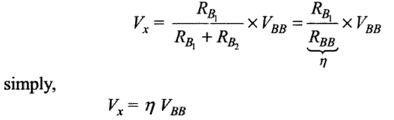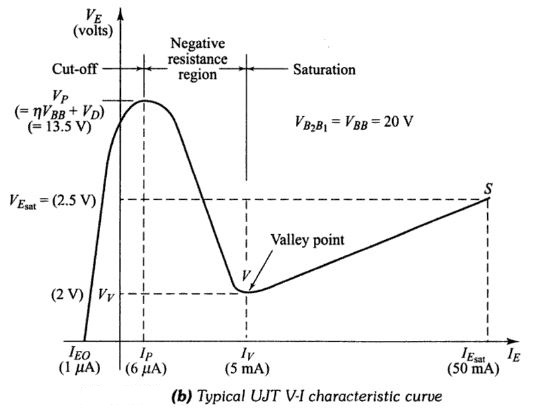# Unijunction Transistor (UJT) With Operation & Applications

#### What Is Unijunction Transistor (UJT)?

The unijunction transistor, abbreviated UJT, is a three-terminal, single-junction device. The basic UJT and its variations are essentially latching switches whose operation is similar to the four-layer diode, the most significant difference being that the UJT’s switching voltage can be easily varied by the circuit designer. Like the four-layer diode, the UJT is always operated as a switch and finds most frequent applications in oscillators, timing circuits, and SCR/TRIAC trigger circuits.

### Unijunction Transistor (UJT) Operation

A typical UJT structure, pictured in the figure, consists of a lightly doped, N-type silicon bar provided with ohmic contacts at each end. The two end connections are called base-l , designated B, and base-2, B2. A small, heavily doped P-region is alloyed into one side of the bar closer to 82. This P-region is the UJT emitter E, and forms a P-N junction with the bar.An InterBase resistance, RBB, exists between B1 and B2. It is typically between 4 kΩ and 10kΩ, and can easily be measured with an ohmeter with the emitter open. RBB is essentially the resistance of the N-type bar. This interbase resistance can be broken up into two resistances, the resistance from B1 to the emitter, called RB1 and resistance from B2 to the emitter, called RB2. Since the emitter is closer to B2, the value of RB1 is greater than RB2 (typically 4.2 kΩ versus 2.8 kΩ).
The operation of the UJT can better be explained with the aid of an equivalent circuit.The UJT’s circuit symbol and its equivalent circuit are shown in below. The diode represents the P-N junction between the emitter and the base-bar (point x). The arrow through RB1, indicates that it is variable since during normal operation it may typically range from 4 kΩ down to 10 Ω.#### The essence of UJT operation can be stated as follows:

(a) When the emitter diode is reverse biased, only a very small emitter current flows. Under this condition, RB1 is at its normal high-value (typically 4 kΩ). This is the UJT’s “off” state.
(b) When the emitter diode becomes forward biased, RB1 drops to a very low value (reason to be explained later) so that the total resistance between E and BI becomes very low, allowing emitter current to flow readily. This is the “on” state.

### Circuit Operation of Unijunction Transistor (UJT)

The UJT is normally operated with both B2 and E biased positive relative to B1 as shown in below figure. B1 is always the UJT reference terminal and all voltages are measured relative to B1. The VBB source is generally fixed and provides a constant voltage from B2 to B1. The VEE source is generally a variable voltage and is considered the input to the circuit. Very often, VEE is not a source but a voltage across a capacitor.We will analyze the UJT circuit operation with the aid of the UJT equivalent circuit, shown inside the dotted lines in Fig.(a). We will also utilize the UJT emitter-base-1 VE-IE curve shown in Fig.(b). The curve represents the variation of emitter current  IE, with emitter-base-1 voltage, VE, at a constant B2-B1 voltage. The important points on the curve are labeled, and typical values are given in parentheses.
The “Off ” state If we neglect the diode for a moment, we can see in Fig.(a) that RB1 and RB2 form a voltage divider that produces a voltage Vx, from point x relative to the ground.Where η (the Greek letter “eta”) is the internal UJT voltage divider ratio RB1/RBB and is called the intrinsic standoff ratio.
Values of η typically range from 0.5 to 0.8 but are relatively constant for a given UJT.
The voltage at point x is the voltage on the N-side of the P-N junction. The VEE source is applied to the emitter which is the P-side. Thus, the emitter diode will be reverse-biased as long as VEE is less than Vx This is the “off” state and is shown on the VE-IE curve as being a very low current region. In the “off” state, then, we can say that the UJT has a very high resistance between E and B1, and IE is usually a negligible reverse leakage current. With no IE, the drop across RE is zero and the emitter voltage, VE, equals the source voltage.
The UJT “off ” state, as shown on the VE-IE curve, actually extends to the point where the emitter voltage exceeds Vx by the diode threshold voltage, VD, which is needed to produce the forward current through the diode. The emitter voltage and this point, P, is called the peak-point voltage, VP, and is given by
VP= Vx + VD= ηVBB+ VD
where VD is typically 0.5 V. For example, if η = 0.65 and VBB= 20V, then VP=13.5 V. Clearly, VP will vary as VBB varies.
The “On ” state As VEE increases, the UJT stays “off” until VE approaches the peak-point value VP, then things begin to happen. As VE approaches Vp, the P-N junction becomes forward biased and begins to conduct in the opposite direction.Note on the VE-IE curve that IE becomes positive near the peak point P. When VE exactly equals VP, the emitter current equals IP, the peak-point current. At this point, holes from the heavily doped emitter are injected into the N-type bar, especially into the B1 region. The bar, which is lightly doped, offers very little chance for these holes to recombine. As such, the lower half of the bar becomes replete with additional current carriers (holes) and its resistance RB1, is drastically reduced. The decrease in RB1 causes Vx to drop. This drop, in turn causes the diode to become more forward biased, and IE increases even further. The larger IE injects more holes into B1further reducing RB1, and so on. When this regenerative or snowballing process ends, RB1. has dropped to a very small value (2-25 Ω) and IE can become very large, limited mainly by external resistance RE.The UJT operation has switched to the low-voltage. the high-current region of its VE- IE curve. The slope of this “on” region is very steep, indicating a low resistance. In this region, the emitter voltage VE, will be relatively small, typically 2V, and remains fairly constant as IE is increased up to its maximum rated value, IE(sat). Thus, once the UJT is “on,” increasing VEE will serve to increase IE while VE remains around 2V.
Turning “Off” the UJT Once it is “on,” the UJT‘s emitter current depends mainly on VEE and RE. As VEE decreases, IE will decrease along the “on” portion of the VE – IE curve. When IE decreases to point V, the valley point, the emitter current is equal to IV, the valley current, which is essentially the holding current needed to keep the UJT “on”. When 15 is decreased below IV, the UJT turns “off” and its operation rapidly switches back to the “off” region of its VE – IE curve, where IE = 0 and VE – VEE. The valley current is the counterpart of the holding current in PNPN devices and generally ranges between 1 and 10 mA.

### Applications of UJT:

Unijunction transistors are used extensively in oscillator, pulse and voltage sensing circuits. Some of the important applications of UJT are discussed below :
(i) UJT relaxation oscillator.
(ii) Overvoltage detector.